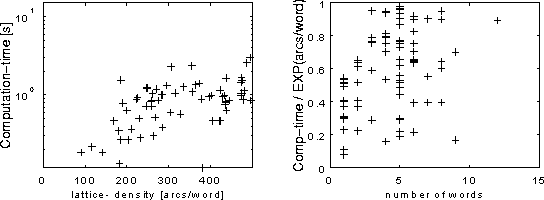Proc. IEEE Workshop on ASR '95, Snowbird, Utah, pp. 125-126.

# Generation and Minimization of Word Graphs in Continuous Speech Recognition

Department of Speech, Music and Hearing, KTH, Stockholm, Sweden

The ability of an automatic speech recognition system (ASR) to output multiple recognition hypotheses is becoming increasingly important. For example in dialogue systems and machine translation systems where the ASR must interact with modules that handle discourse, semantics and pragmatics. Passing multiple recognition hypotheses to the higher levels of the system is a way of increasing the coupling without losing the modularity, which is essential for the development of large complex systems. Another application is hypothesis re-scoring, where the multiple hypotheses are an internal, intermediate representation generated by a fast initial search. They are then used to limit the search-space in a second, more accurate search.

Recently , representations of multiple hypotheses in the form of graphs or lattices have been explored. Representing the hypotheses in an acyclic graph instead of a tree or an n-best list opens the possibility to move from algorithms using amount of time and space that are exponential functions of the input length, to algorithms of polynomial and even linear complexity. The latter is of course the desired complexity class for an algorithm intended for use in a real-time system.

In this study, word graphs where generated in a two-pass search strategy similar to the algorithm of . The search is based on the tree-trellis A* algorithm , but the second, stack-decoding pass is modified to build an acyclic graph instead of a tree. This has the advantage that the graph can be constructed in time linear with respect to the input length which is clearly the optimal computational complexity class. Also, because we use identical models in the forward and backward passes, it is guaranteed that the generated graph is the minimal word-lattice, containing exactly the paths that have a higher score than the threshold. This includes all the different alignments of each word-string.

For re-scoring using new acoustical models, the word-lattice constructed above is the optimal minimal representation because it is desirable to re-score the different alignments. However, for re-scoring using only a new grammar, a more compact representation is better. Minimizing a word-lattice is equivalent to minimizing a non-deterministic finite-state automaton (NFA) which is a hard problem that can not in general be solved in polynomial time. Therefore, the problem has been attacked using heuristic methods that reduce the graph but not to the minimal size. In particular, the so called word-pair approximation has been applied .

In this study we instead approached the problem by applying the classical algorithms for: 1) constructing an equivalent deterministic finite automaton (DFA) and 2) minimizing of the DFA to obtain the minimal deterministic graph . Because of the structure of word-graphs, the minimization can be done in linear time. Constructing the DFA is harder and in general not possible to perform in polynomial time with respect to the graph-size. However, the graph-size has at least two dimensions: the utterance-length and the graph-density (number of arcs / utterance-length) . Graph-density corresponds to the amount of pruning and utterance-length corresponds to the length of the input.

We empirically studied the time-complexity of the construction of the DFA in the 1000-word spontaneous speech task of the Waxholm-project . In the left graph of Figure 1 we see the exponential behavior with respect to lattice-density, but the right graph shows approximately linear time-dependence with respect to utterance-length when the dependency of the graph-density have been eliminated.

For comparison, the word-pair approximation was also investigated. Applying the word-pair approximation to the word-lattice resulted on average in a reduction in the number of arcs to 8.1% of the lattice. The minimal deterministic graph for the same word-lattice was on average reduced to 1.4% of the lattice. In contrast to the word-pair approximation, the graph of the proposed algorithm generates exactly the same word-strings as the original lattice (no hypotheses are lost by approximation). However, since the proposed method is exponential with respect to lattice-density, a hybrid-approach, where the lattice is first reduced by the word-pair approximation and then minimized, seems to be an attractive alternative.Figure 1. Left: Time for construction of the DFA as a function of the lattice-density. Right: Construction time divided by the exponential of the lattice density. The simulation was made on a SPARC 10 workstation.

## References

 Hetherington, Phillips, Glass & Zue (1993) : "A* Word Network Search for Continuous Speech Recognition", ICASSP '93, pp 1533-1536.

 Murveit, Butzberger, Digalakis & Weintraub (1993) : "Large-Vocabulary Dictation Using SRI's Decipher Speech Recognition System: Progressive Search Techniques", ICASSP '93, pp II-319-322.

 Soong & Huang (1991) : "A Tree-Trellis Based Fast Search for Finding the N Best Sentence Hypotheses in Continuous Speech Recognition", ICASSP '91, pp 713-716.

 Blomberg, Carlson, Elenius, Granström, Gustafson, Hunnicut, Lindell & Neovius (1993) : "An Experimental Dialogue System: Waxholm", EUROSPEECH '93, pp 1867-1870.

 Ström (1994) : "Optimising the lexical representation to improve A* lexical search", STL-QPSR 2-3/1994, pp 113-124. (Abstract) (Paper, postscript 225K)

 Ney & Aubert (1994) : "A Word Graph Algorithm for Large Vocabulary, Continuous Speech Recognition", ICSLP '94, pp 1355-1358.

 Hopcroft & Ullman (1979) : Introduction to automata theory, languages and computation, Addison & Wesley, ISBN 0-201-02988X.